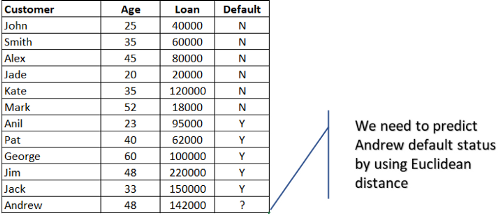top of page
Search
• Dhilip Subramanian

# A Simple Introduction to K-Nearest Neighbours Algorithm## What is KNN?K Nearest Neighbour is a simple algorithm that stores all the available cases and classifies the new data or case based on a similarity measure. It is mostly used to classifies a data point based on how its neighbours are classified.

Let’s take below wine example. Two chemical components called Rutime and Myricetin. Consider a measurement of Rutine vs Myricetin level with two data points, Red and White wines. They have tested and where then fall on that graph based on how much Rutine and how much Myricetin chemical content present in the wines.‘k’ in KNN is a parameter that refers to the number of nearest neighbours to include in the majority of the voting process.

Suppose, if we add a new glass of wine in the dataset. We would like to know whether the new wine is red or white?So, we need to find out what the neighbours are in this case. Let’s say k = 5 and the new data point is classified by the majority of votes from its five neighbours and the new point would be classified as red since four out of five neighbours are red.## How shall I choose the value of k in KNN Algorithm?‘k’ in KNN algorithm is based on feature similarity choosing the right value of K is a process called parameter tuning and is important for better accuracy. Finding the value of k is not easy.

## Few ideas on picking a value for ‘K’

1) Firstly, there is no physical or biological way to determine the best value for “K”, so we have to try out a few values before settling on one. We can do this by pretending part of the training data is “unknown”

2) Small values for K can be noisy and subject to the effects of outliers.

3) Larger values of K will have smoother decision boundaries which mean lower variance but increased bias.

4) Another way to choose K is though cross-validation. One way to select the cross-validation dataset from the training dataset. Take the small portion from the training dataset and call it a validation dataset, and then use the same to evaluate different possible values of K. This way we are going to predict the label for every instance in the validation set using with K equals to 1, K equals to 2, K equals to 3.. and then we look at what value of K gives us the best performance on the validation set and then we can take that value and use that as the final setting of our algorithm so we are minimising the validation error.

5) In general, practice, choosing the value of k is k = sqrt(N) where N stands for the number of samples in your training dataset.

6) Try and keep the value of k odd in order to avoid confusion between two classes of data

## How does KNN Algorithm works?

In the classification setting, the K-nearest neighbor algorithm essentially boils down to forming a majority vote between the K most similar instances to a given “unseen” observation.

Similarity is defined according to a distance metric between two data points. A popular one is the Euclidean distance method.Other methods are Manhattan, Minkowski, and Hamming distance methods.

For categorical variables, the hamming distance must be used.

Let’s take a small example. Age vs loan.We need to predict default status for Andrew (Yes or No).

Calculate Euclidean distance for all the data points.With K=5, there are two Default=N and three Default=Y out of five closest neighbors. We can say default status for Andrew is ‘Y’ based on the major similarity of 3 points out of 5.

K-NN is also a lazy learner because it doesn’t learn a discriminative function from the training data but “memorises” the training dataset instead.

## Pros of KNN

1. Simple to implement

2. Flexible to feature/distance choices

3. Naturally handles multi-class cases

4. Can do well in practice with enough representative data

## Cons of KNN

1. Need to determine the value of parameter K (number of nearest neighbours)

2. Computation cost is quite high because we need to compute the distance of each query instance to all training samples.

3. Storage of data

4. Must know we have a meaningful distance function.